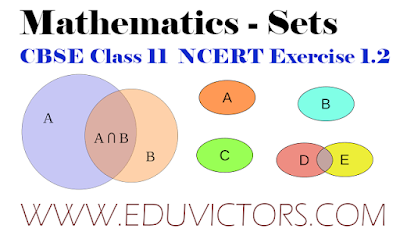## NCERT Exercise 1.2

Q1. Which of the following are examples of the null set
(i) Set of odd natural numbers divisible by 2

(ii) Set of even prime numbers

(iii) { x : x is a natural numbers, x < 5 and x > 7 }

(iv) { y : y is a point common to any two parallel lines}

Q2. Which of the following sets are finite or infinite
(i) The set of months of a year

(ii) {1, 2, 3, . . .}

(iii) {1, 2, 3, . . .99, 100}

(iv) The set of positive integers greater than 100

(v) The set of prime numbers less than 99

Q3. State whether each of the following set is finite or infinite:
(i) The set of lines which are parallel to the x-axis

(ii) The set of letters in the English alphabet

(iii) The set of numbers which are multiple of 5

(iv) The set of animals living on the earth

(v) The set of circles passing through the origin (0,0)

Q4. In the following, state whether A = B or not:
(i) A = { a, b, c, d } B = { d, c, b, a }

(ii) A = { 4, 8, 12, 16 } B = { 8, 4, 16, 18}

(iii) A = {2, 4, 6, 8, 10} B = { x : x is positive even integer and x ∈ 10}

(iv) A = { x : x is a multiple of 10}, B = { 10, 15, 20, 25, 30, . . . }

Q5. Are the following pair of sets equal ? Give reasons.
(i) A = {2, 3}, B = {x : x is solution of x² + 5x + 6 = 0}

Answer: x² + 5x + 6 = 0
⇒ x² + 3x + 2x + 6 = 0
⇒ (x + 2)(x + 3) = 0
⇒ x = -2  or  x = -3

Clearly, A ≠ B

(ii) A = { x : x is a letter in the word FOLLOW}
B = { y : y is a letter in the word WOLF}

A = { x : x is a letter in the word FOLLOW} = {F, O, L, W}
B = { y : y is a letter in the word WOLF} = {W, O, L, F}

Since every member of set A is in B and every element of set B is in A.
⇒ A = B

Q6. From the sets given below, select equal sets :
A = { 2, 4, 8, 12},
B = { 1, 2, 3, 4},
C = { 4, 8, 12, 14},
D = { 3, 1, 4, 2}
E = {–1, 1},
F = { 0, a},
G = {1, –1},
H = { 0, 1}

Clearly n(G) = n(E) = n(H) = n(F) = 2
∴ Sets G, E, H and F are equivalent sets.

Also n(A) = n(B) = n(C) = n(D) = 4
∴ Sets A, B, C and D are equivalent sets.

Since every element of B is in D, and every element of D is in B.
∴ D = B

Since every element of E is in G, and every element of G is in E.
∴ E = G